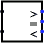#Comparator

 Library: Arithmetic Introduced: 2.0 Beta 22 Appearance:## Behavior

Compares two values, either as unsigned values or as two's-complement values, depending on the Numeric Type attribute. Normally, one of the outputs will be 1, and the other two outputs will be 0.

The comparison is performed starting at the most significant bits in each number and descending downward in parallel until a location is found where the two values disagree. If, however, an error value or a floating value is encountered during this descent, then all outputs will match that error or floating value.

## Pins

West edge, north end (input, bit width matches Bit Width attribute)
The first of the two values to be compared.
West edge, south end (input, bit width matches Bit Width attribute)
The second of the two values to be compared.
East edge, labeled > (output, bit width 1)
1 if the first input is greater than the second input, 0 if the first input is less than or equal the second input.
East edge, labeled = (output, bit width 1)
1 if the first input equals the second input, 0 if the first input is not equal the second input.
East edge, labeled < (output, bit width 1)
1 if the first input is less than the second input, 0 if the first input is greater than or equal the second input.

## Attributes

Data Bits
The bit width of the component's inputs and outputs.

None.

## Text Tool Behavior

None.

Back to Library Reference